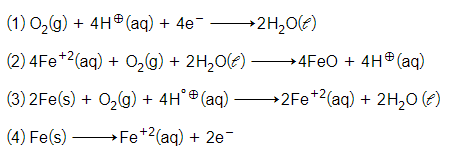the emf of Voltaic cell

(1) 1.25 V

(2) -1.75 V

(3) 1.75 V

(4) 4 V

Concept Questions :-

Nernst Equation
High Yielding Test Series + Question Bank - NEET 2020

Difficulty Level:

The same amount of electric current is apassed through aqueous solution of MgSO4 and AlCl3. If 2.8 g Mg metal is deposited at amount of Al metal deposited in second cell will be

(1) 2.1 g

(2) 2.49 g

(3) 3.15 g

(4) 3.73g

Concept Questions :-

High Yielding Test Series + Question Bank - NEET 2020

Difficulty Level:

The potential of following cell at K is-

(1) -0.118V

(2) -0.0591V

(3) 0.118V

(4) 0.0591V

Concept Questions :-

Nernst Equation
High Yielding Test Series + Question Bank - NEET 2020

Difficulty Level:

Select the incorrect statement for dry cell

(1) Mn is reduced from Mn+4 to Mn+3

(2) NH3 gas libreted out

(3) Zn is used as anode

(4) Paste of NH4Cl and ZnCl2 is used

Concept Questions :-

Electrochemical series
High Yielding Test Series + Question Bank - NEET 2020

Difficulty Level:

What is the current efficiency of an electrode deposition of Cu metal from CuSO4 solution in which 9.8 gm copper is deposited by the passage of 5 amperes current for 2 hours?

1. 41.4 %

2. 50%

3. 75%

4. 82.8 %

Concept Questions :-

High Yielding Test Series + Question Bank - NEET 2020

Difficulty Level:

EMF of the following cell will be zero if

Pt(H2)|H+||H+|(H2)Pt

P1   C1  C2    P2

(1) C1P1 = C2P2

(2) C1P2 = P2C1

(3)

(4) None of these

Concept Questions :-

Nernst Equation
High Yielding Test Series + Question Bank - NEET 2020

Difficulty Level:

For the cell reaction

the change in free energy at a given temperature is a function of

(1) $\mathcal{l}$nC1

(2) $\mathcal{l}$n$\frac{{\mathrm{C}}_{2}}{{\mathrm{C}}_{1}}$

(3) $\mathcal{l}$n (C1 +C2)

(4) $\mathcal{l}$nC2

Concept Questions :-

Electrode Potential / Introduction
High Yielding Test Series + Question Bank - NEET 2020

Difficulty Level:

Which of the following is an incorrect statement :-

(1) Mercury cell is a primary cell providing a constant potential

(2) During recharging lead storage cell works as electrolytic cell

(3) Galvanised iron does not rust

(4) In electric cell reduction occurs at anode and in galvanic cell oxidation takes place at anode

Concept Questions :-

Electrochemical series
High Yielding Test Series + Question Bank - NEET 2020

Difficulty Level:

The cell reaction of micro electrochemical cell fromed during rusting of iron is:-Concept Questions :-

Corrosion
High Yielding Test Series + Question Bank - NEET 2020

Difficulty Level:

Electrolysis of hot aqueous solution of NaCl gives NaClO4 as-

How many faraday are required to obtain 1000 g of sodium perchlorate ?

(1) 66 F

(2) 122.5 F

(3) 15.13 F

(4) 1.86 F

Concept Questions :-